### Home > CALC > Chapter 8 > Lesson 8.2.1 > Problem8-68

8-68.
1. Examine the integrals below. Consider the multiple tools available for evaluating integrals and use the best strategy for each. After evaluating the integral, write a short description of your method. Homework Help ✎

1.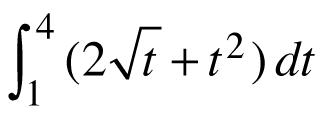2.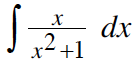3.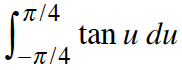4.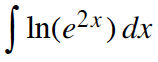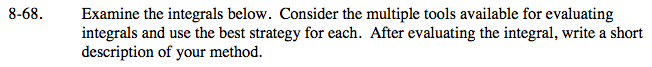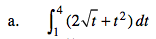Before integrating, rewrite the integrand using a fractional exponent.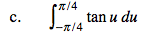Think about the shape of this graph, does it have any symmetry?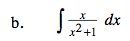Let U = x2 + 1.

Because y = x2 + 1 is always positive, this solution will not need an absolute value sign.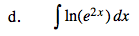Before integrating, use properties of logarithms to rewrite the integrand.

$=\int2xdx=x^2+C$